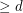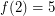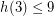# Cube-Simplex conjecture

 Importance: High ✭✭✭
 Author(s): Kalai, Gil
 Subject: Geometry » Polytopes
 Keywords: cube facet polytope simplex
 Posted by: mdevos on: May 9th, 2008
Conjecture   For every positive integer, there exists an integerso that every polytope of dimensionhas a-dimensional face which is either a simplex or is combinatorially isomorphic to a-dimensional cube.

It is an easy consequence of Euler's formula that every 3-polytope has a face which is either a triangle, a quadrilateral, or a pentagon. The 120-cell is a 4-polytope in which every 2-face is a pentagon (in fact every 3-face is a regular dodecahedron). Perles and Shephard asked whether there exist higher dimensional polytopes in which all 2-faces have at least 5 vertices. This question was answered in the negative by Kalai [K] who showed that every 5-polytope has a 2-face with at most 4 vertices. So, if we defineto be the smallest integersatisfying the above conjecture for, orif none exists, then.

This conjecture is still open for simple polytopes. However, it is known that for every positive integer, there exists an integerso that every simple polytope of dimensioneither has a 2-dimensional face which is a triangle, or a-dimensional face which is combinatorially isomorphic to a cube. This was proved by Kalai [K] using some earlier results of Nikulin and of Blind and Blind. Actually, something much stronger holds here: simple polytopes of sufficiently high dimension without 2-faces which are triangles must have most-dimensional faces combinatorially isomorphic to the-cube.

The following is an interesting weakening of the above conjecture.

Conjecture   For every positive integer, there exists an integerand a finite listof-dimensional polytopes, so that every polytope of dimensionhas a-dimensional face which appears in.

Definingto be the smallest integersatisfying this conjecture for, orif none exists, we find that(by the consequence of Euler's formula in the first paragraph). Meisinger, Kleinschmidt, and Kalai [MKK] proved thatwith the help of FLAGTOOL, a computer program which can compute linear relations for-vectors. This weaker conjecture is known to be true for simple polytopes.

## Bibliography

[MKK] G. Meisinger, P. Kleinschmidt, and G. Kalai, Three theorems, with computer-aided proofs, on three-dimensional faces and quotients of polytopes. The Branko Grünbaum birthday issue. Discrete Comput. Geom. 24 (2000), no. 2-3, 413--420. MathSciNet

*[K] G. Kalai, On low-dimensional faces that high-dimensional polytopes must have. Combinatorica 10 (1990), no. 3, 271--280. MathSciNet

* indicates original appearance(s) of problem.